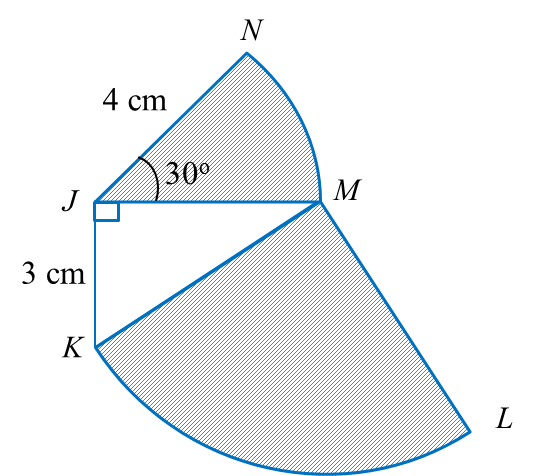# SPM Mathematics (Model Test Paper), Question 7 – 9

SPM Mathematics (Model Test Paper)

Section A
(52 marks)
Answer all questions in this section.

7.   Diagram 4 shows a straight line ST and a straight line PQ drawn on a Cartesian plane.  ST is  parallel to PQ. Given that equation of the straight line ST is 2y = 8x + 3.Diagram 4

Find,
(a)  the equation of the straight line PQ,
(b)  the x-intercept of the straight line PQ.
[ 5 marks ]
(a)
$\begin{array}{l}2y=8x+3\\ y=4x+\frac{3}{2}\end{array}$
m = 4
4 = 4 (6) + c
c = 2
Using formula, y = mx + c
equation of the straight line PQ is y = 4x 20.

(b)
y = 4x 20, at x-intercept, y = 0
4x – 20 = 0,   y= 0
x = 5   or   x-intercept = 5

8.   Diagram 5, shows a quadrant KLM with centre  M and sector JMN with centre J.Diagram 5

Using $\pi =\frac{22}{7}$ , calculate
(a) the perimeter, in cm, of the whole diagram,
(b) the area, in cm2, of the shaded region.
[6 marks]
(a)
KM2 = JK2 + JM2
KM2 = 32+ 42
KM2 = 25
KM = 5 cm
Perimeter of the whole diagram
= NJ + JK + KL+ LM + MN
$\begin{array}{l}=4+3+\left(\frac{1}{4}×2×\frac{22}{7}×5\right)+5+\left(\frac{30}{360}×2×\frac{22}{7}×4\right)\\ =7+\left(\frac{55}{7}\right)+5+\left(\frac{44}{21}\right)\\ =21\frac{20}{21}\text{}cm\end{array}$

(b)
= Area of KLM + Area of JMN
$\begin{array}{l}=\left(\frac{1}{4}×\frac{22}{7}×{5}^{2}\right)+\left(\frac{30}{360}×\frac{22}{7}×{4}^{2}\right)\\ =\frac{274}{14}+\frac{88}{21}\\ =23\frac{16}{21}\text{}c{m}^{2}\end{array}$

9.  Diagram 6 shows the speed-time graph for the movement of two particles, J and K, for a period of t s. The graph ABCD represents the movement of J and the graph AE represents the movement of K. Both particles start at the same point and move along the same route.Diagram 6

(a) State the uniform speed, in ms-1, of particle J.
(b) Calculate the rate of change of speed, in ms-2, of particle J for the first 13 s.
(c) At t s, the difference between the distance travelled by J and K is 169 m. Calculate the value of t.
[ 6 marks ]
(a)
Uniform speed of particle J = 26 ms-1

(b)
Rate of change of speed of particle J for the first 13 s
$=\frac{26-0}{13-0}=2m{s}^{-2}$

(c)
Given at t s, the difference between the distance travelled by J and K is 169
(distance travelled by particle J) – (distance travelled by particle K) = 169
[ ½ (t – 13 + t) × 26 ] – [ ½ (26) (t )] = 169
[ 13 (2t – 13) ] – 13t  = 169
( 26t – 169 – 13t ) = 169
13t  = 338
t  = 26

### 1 thought on “SPM Mathematics (Model Test Paper), Question 7 – 9”

1.very useful..thank you very much 🙂